# Understanding the electric field of a sphere with a hole

baseballfan_ny
Homework Statement:
An insulating sphere of radius R1 has charge density p (rho) uniform, except for a small, hollow region of radius R2 located at a distance a from the center. Find the electric field at a point P inside the hollow region.
Relevant Equations:
Gauss' Law, superposition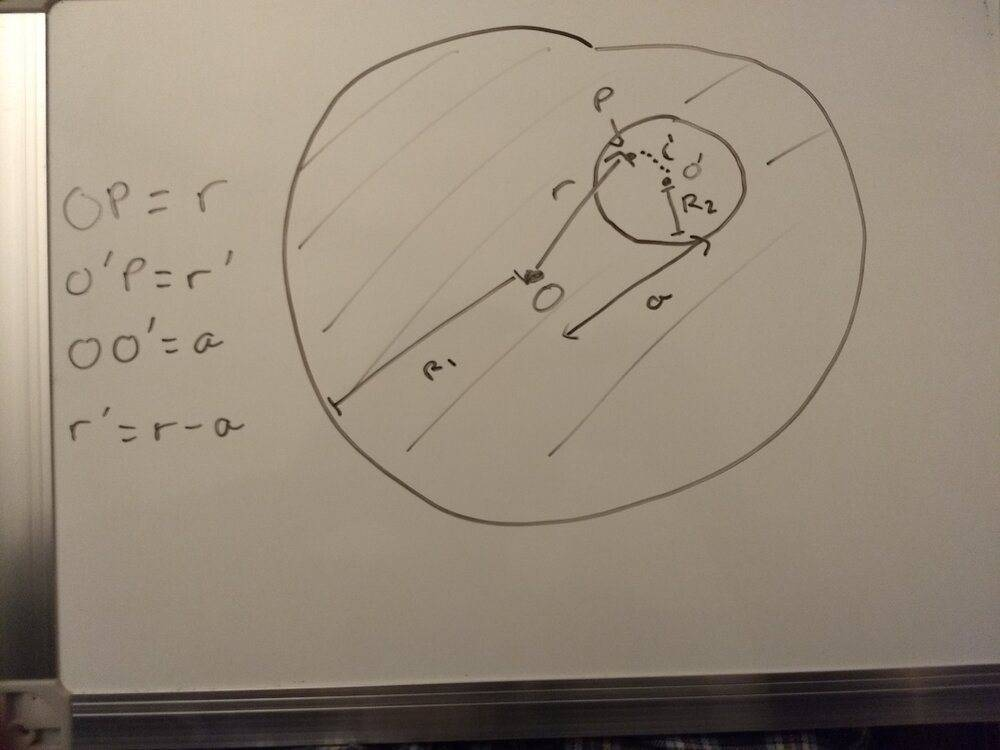Here's an image. O and O' are the respective centers, a is the distance between them, r is the distance from the center of the sphere to P, and r' = r - a, the distance from O' to P.

The approach (which I don't understnad) given is to use Gauss' Law and superposition, so that we calculate the electric field at P if there was no hollow sphere and get,
##\iint_{Closed Surface} \vec E \cdot d\vec A = \frac {Q_{enc}} {\epsilon_0}##
## E 4\pi*r^2 = \frac {\rho * \frac {4} {3} \pi*r^3} {\epsilon_0}##
## E = \frac {\rho r} {3\epsilon_0}##

Then the hollow sphere is treated as a region of "negative charge density" ##\rho##, and we calculate E at P from O' (at a distance r'), so we get ##E = -\frac {\rho r'} {3\epsilon_0}##

And we add the two together to find ##E_P = \frac {\rho r - r'} {3\epsilon_0} = \frac {\rho a} {3\epsilon_0}##.

This approach really confuses me and I am not sure why it works. I get that we have to subtract some charge out given that there's an empty sphere, but I do not understand why we do so with a sphere of radius r' around the center of the hollow sphere. For some clarification, to me it looks like that when I draw a Gaussian surface through Point P, there's not enough symmetry to apply Gauss' Law, as shown in the picture below: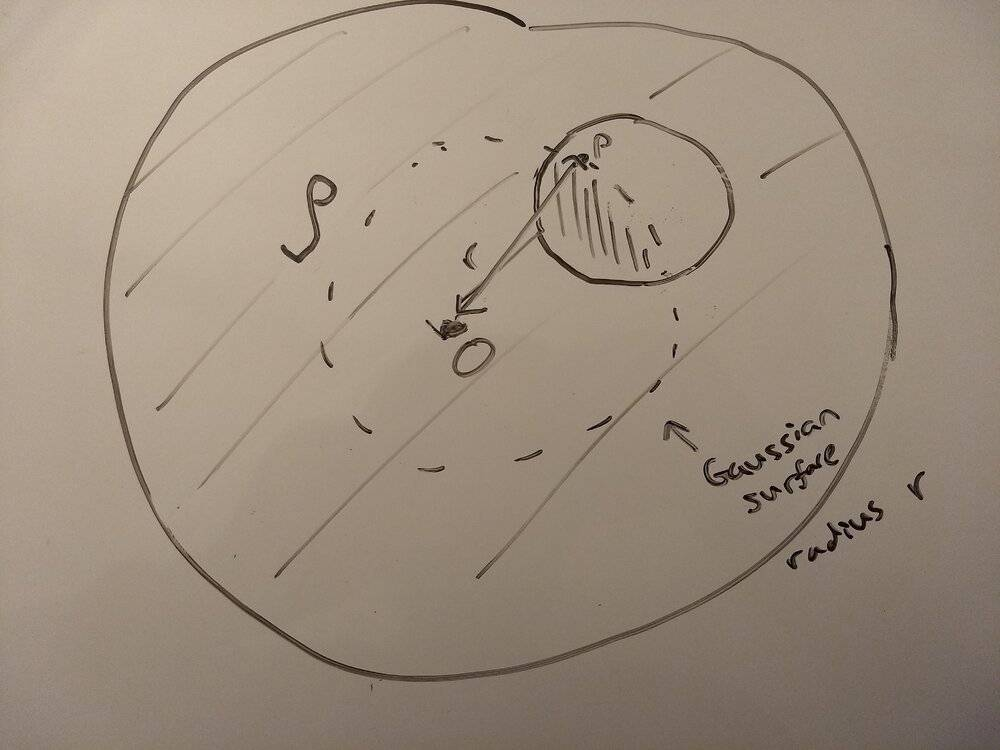Then I would assume we need to subtract the region I've shaded in above (the intersection between the Gaussian surface and the hollow sphere), as that's the enclosed region where there's "missing charge" and is causing the "disruption in symmetry." But the approach is to instead subtract a region of radius r' from the center of the hollow sphere, which is the shaded region illustrated in the image below: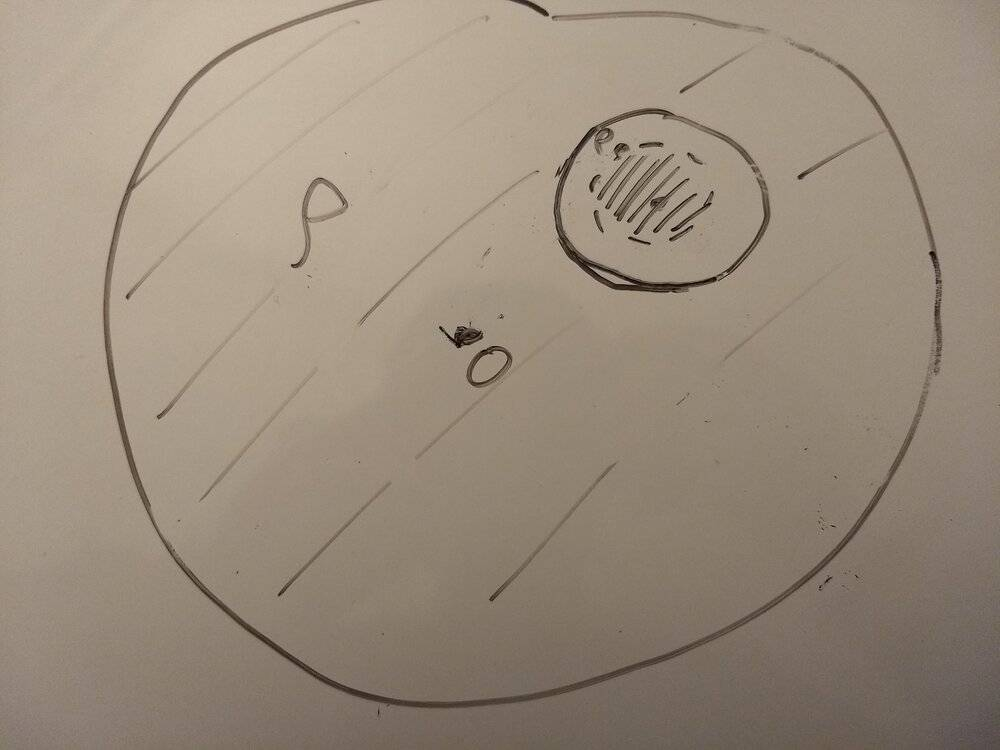I really am having trouble understanding why we have to subtract this region rather than the previous one. Are the two geometrically equivalent? Or am I totally missing something about satisfying the symmetry for the Divergence Theorem?

Thank you so much in advance!

•Delta2

Homework Helper
Gold Member
Consider the sphere of radius R1 without the cavity. You can imagine the sphere as made up of region I and region II as shown: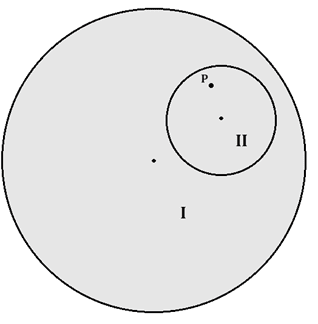Region II is where the cavity would be located if we had the cavity; but, we want to imagine, for now, that there is no cavity.

This sphere has enough symmetry that you can apply Gauss' law to find ##\vec E_{\rm tot}## at point P due to this sphere (which has no cavity).

By the principle of superposition, ##\vec E_{\rm tot} = \vec E_{\rm I} + \vec E_{\rm II}## , where ##\vec E_{\rm I}## is the field at P due to just the charge in region I and ##\vec E_{\rm II}## is the field at P due to just the charge in region II. Which term in this equation represents the field at point P when the cavity is present?

•Delta2, etotheipi, baseballfan_ny and 1 other person
Homework Helper
Gold Member
Perhaps you are confused about how superposition works. It's really simple. You do two separate calculations. One for the positively-charged green solid sphere (see figure) at point P1 and another for the negatively-charged blue solid sphere at point P2. These fields at the two points exist independently of one another.
Study the figure below. When you bring the spheres together (superimpose), the field at point P would be the sum ##\vec E_1+\vec E_2## of the separate solid spheres at that point. Also, the charge in the white spherical region on the right is zero because you have as much positive charge as you have negative. It's as simple as that.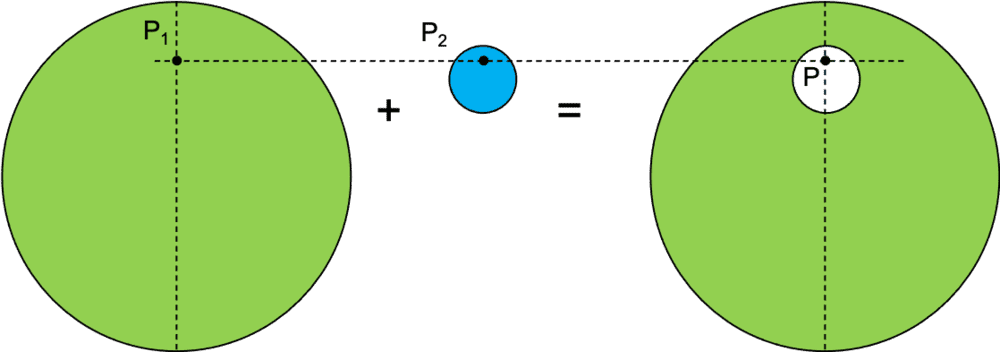Edit: Rats! @TSny beat me to the punch.

•Delta2, baseballfan_ny, TSny and 1 other person
Homework Helper
Gold Member
Edit: Rats! @TSny beat me to the punch.
Close...by about 2 seconds•SammyS
baseballfan_ny
Thank you both so much for the responses!

I think I'm getting there but I'm still kind of confused.

This sphere has enough symmetry that you can apply Gauss' law to find E→tot at point P due to this sphere (which has no cavity).

By the principle of superposition, E→tot=E→I+E→II , where E→I is the field at P due to just the charge in region I and E→II is the field at P due to just the charge in region II. Which term in this equation represents the field at point P when the cavity is present?

Well I guess based on the technique ##\vec E_{tot} ## is the field when the cavity is present but, now ##\vec E_{II}## has to have the opposite sign/direction? I still don't get why this works. Maybe I'm thinking too much in terms of Gauss' Law? If we consider the image you posted above in the situation where there's no cavity, how is ##\vec E_{tot} ## representing the electric field at point P? Is ##\vec E_{I}## due to all the charge enclosed by a Gaussian surface in region I up to Point P (minus that in region II)? And then ##\vec E_{II}## is due to all the charge enclosed by a Gaussian surface from the center of region II up to Point P? (like the last two drawing I have in my original post). How do these add to give the same result as just taking a Gaussian surface through Point P?

Study the figure below. When you bring the spheres together (superimpose), the field at point P would be the sum E→1+E→2 of the separate solid spheres at that point. Also, the charge in the white spherical region on the right is zero because you have as much positive charge as you have negative. It's as simple as that.

I guess this makes sense. What I'm still kind of confused about is how superimposing a sphere up to P from the center of the large sphere with a sphere up to P from the center of the cavity is equivalent to a gaussian sphere from the center of the large sphere up to P with all the charge in the cavity subtracted?

•Delta2
Homework Helper
Gold Member
Well I guess based on the technique ##\vec E_{tot} ## is the field when the cavity is present
No. ##\vec E_{\rm tot}## is the electric field that would be at point P if there is no cavity. ##\vec E_{\rm tot}## is easy to find using Gauss's law.

##\vec E_{\rm tot}## is not the answer to the question since you want the field at P when the cavity is present. However, the trick is to realize that, according to the superposition principle, ##\vec E_{\rm tot} = \vec E_{\rm I} +\vec E_{\rm II}##. One of the terms on the right side represents the answer to the question. The other term on the right is easy to find using Gauss's law.

•Delta2 and baseballfan_ny
Homework Helper
Gold Member
I guess this makes sense. What I'm still kind of confused about is how superimposing a sphere up to P from the center of the large sphere with a sphere up to P from the center of the cavity is equivalent to a gaussian sphere from the center of the large sphere up to P with all the charge in the cavity subtracted?
You do not use Gauss's law to find the field for the sphere with the cavity. You use it twice to find the fields ##\vec E_1## and ##\vec E_2## at points P1 and P2 for the solid spheres. Then you say, "Lookee here! If I add the two fields together as vectors, I should the field at point P of the solid sphere with the cavity." That's because the charge distribution in space of the smaller negative solid sphere inside the larger positive sphere is identical to the charge distribution of a positive sphere with a cavity. Since the electric field depends only on the charge distribution, the superposition of ##\vec E_1## and ##\vec E_2## should be the required field.

•baseballfan_ny
baseballfan_ny
No. ##\vec E_{\rm tot}## is the electric field that would be at point P if there is no cavity. ##\vec E_{\rm tot}## is easy to find using Gauss's law.

##\vec E_{\rm tot}## is not the answer to the question since you want the field at P when the cavity is present. However, the trick is to realize that, according to the superposition principle, ##\vec E_{\rm tot} = \vec E_{\rm I} +\vec E_{\rm II}##. One of the terms on the right side represents the answer to the question. The other term on the right is easy to find using Gauss's law.

Ok. I think I sort of see it now. ##\vec E_{\rm tot}## is the electric field due to just the positively charged sphere, no cavity. And that is the same as summing ##\vec E_{\rm I}## (the electric field with cavity) and ##\vec E_{\rm II}## (the electric field where the Gaussian surface is taken from the center of the cavity to P). And that's the same thing as superposing a negative sphere the size of the cavity with the larger positive sphere.

You do not use Gauss's law to find the field for the sphere with the cavity.

Perhaps this had been messing me up, as I was trying to draw a Gaussian sphere for the large sphere with the cavity and equate it to the superposition of the two spheres, which seemed to give rise to a ton of really confusing geometry. But if I understand, what we're doing here is modeling a sphere with a cavity as two filled spheres with opposite charge, and using Gauss' law to add the field contributions from each at a given point (as y'all said earlier). And that happens to give us a result independent of our position inside the cavity.

And then I suppose at a distance really far away much greater than the radius of the large sphere, it becomes like a particle and you could just apply Gauss' Law without superposition. But (as a secondary question) if you wanted to find the field at a point outside the hollow region but inside the large sphere, would you still use superposition (superposing the field from a filled positive sphere at that point and the field outside of a smaller negative sphere the size of the cavity)?

•Delta2 and TSny
Homework Helper
Gold Member
Ok. I think I sort of see it now. ##\vec E_{\rm tot}## is the electric field due to just the positively charged sphere, no cavity. And that is the same as summing ##\vec E_{\rm I}## (the electric field with cavity) and ##\vec E_{\rm II}## (the electric field where the Gaussian surface is taken from the center of the cavity to P). And that's the same thing as superposing a negative sphere the size of the cavity with the larger positive sphere.
Yes. That's a good description.

•baseballfan_ny
Homework Helper
Gold Member
And then I suppose at a distance really far away much greater than the radius of the large sphere, it becomes like a particle and you could just apply Gauss' Law without superposition. But (as a secondary question) if you wanted to find the field at a point outside the hollow region but inside the large sphere, would you still use superposition (superposing the field from a filled positive sphere at that point and the field outside of a smaller negative sphere the size of the cavity)?
Yes, that's right. The result will not be as simple as for points P inside the cavity.

You could also use this superposition idea to find the field at a point outside the large sphere but in the vicinity of the large sphere.

•baseballfan_ny
baseballfan_ny
Yes, that's right. The result will not be as simple as for points P inside the cavity.

You could also use this superposition idea to find the field at a point outside the large sphere but in the vicinity of the large sphere.

Ok got it, thank you so much! Unrelated question, how do you all make your diagrams for these posts? Are you just editing shapes on Powerpoint or something?

•Delta2
Homework Helper
Gold Member
Ok got it, thank you so much! Unrelated question, how do you all make your diagrams for these posts? Are you just editing shapes on Powerpoint or something?
I use the Paint program in Windows.

•baseballfan_ny and Delta2
•